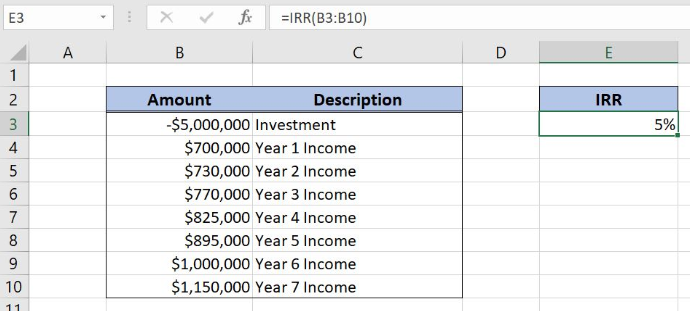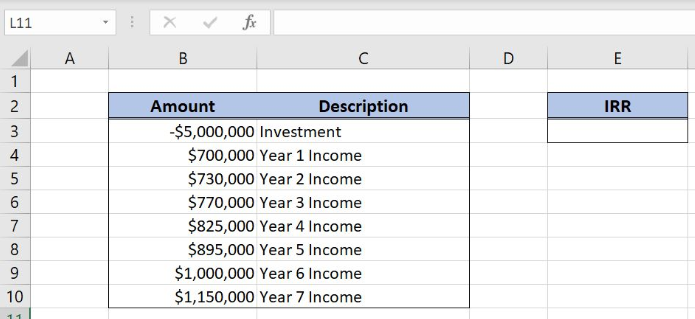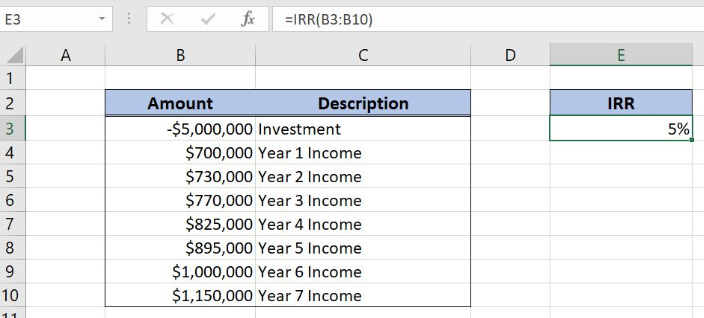Get instant live expert help with Excel or Google Sheets“My Excelchat expert helped me in less than 20 minutes, saving me what would have been 5 hours of work!”

#### Post your problem and you’ll get Expert help in seconds.

Your message must be at least 40 characters
Our professional Expert are available now. Your privacy is guaranteed.

# Excel IRR Function

Excel allows a user to get an internal rate of return of an investment using the IRR function. This step by step tutorial will assist all levels of Excel users in getting an IRR of an investment.Figure 1. The result of the IRR function

## Syntax of the IRR Formula

The generic formula for the IRR function is:

`=IRR (values, [guess])`

The parameter of the IRR function is:

• values – a range of cells containing values, including initial investment and incomes. The investment must have negative sign, as it is a cost
• [guess] – an estimated value for the expected IRR. This parameter is non-mandatory. If it’s omitted, the function will take a default value of 0.1 (=10%).

## Setting up Our Data for the IRR Function

Let’s look at the structure of the data we will use. In column B (“Amount”), we have values including initial investment and yearly incomes. In column C (“Description”) we have description of every amount. In the cell E3, we want to get the IRR.Figure 2. Data that we will use in the IRR example

## Get an IRR of Values Using the IRR Function

In our example, we want to get the IRR of the values in the range B3:B10. The result will be in the cell E3.

The formula looks like:

`=IRR(B3:B10)`

The parameter values is the range B3:B10

To apply the IRR function, we need to follow these steps:

• Select cell E3 and click on it
• Insert the formula: `=IRR(B3:B10)`
• Press enter.Figure 3. Using the IRR function to get the internal rate of the investment

Finally, the result in the cell E3 is 5%, which is the internal rate of the investment.

Most of the time, the problem you will need to solve will be more complex than a simple application of a formula or function. If you want to save hours of research and frustration, try our live Excelchat service! Our Excel Experts are available 24/7 to answer any Excel question you may have. We guarantee a connection within 30 seconds and a customized solution within 20 minutes.

### Did this post not answer your question? Get a solution from connecting with the expert.Another blog reader asked this question today on Excelchat:## Subscribe to Excelchat.coAnother blog reader asked this question today on Excelchat: